• FAQ
• Contact/ Homework Answers / Accounting / 61.Use the appropriate factors from Table 6-4 or Table 6-5
Not my Question
Flag Content

# Question

61.Use the appropriate factors from Table 6-4 or Table 6-5 to answer the following questions.

(a.) What is the present value of \$90,000 to be received in six years using a discount rate of 12%?
(b.) How much should be invested today at a return on investment of 16% compounded annually to have \$150,000 in eleven years?

62.Use the appropriate factors from Table 6-2 or Table 6-3 to answer the following question.

(a.) Vincent Co.'s common stock is expected to have a dividend of \$7 per share for each of the next five years, and it is estimated that the market value per share will be \$65 at the end of five years. If an investor requires a return on investment of 14%, what is the maximum price the investor would be willing to pay for a share of Vincent Co. common stock today?
(b.) Aliyana bought a bond with a face amount of \$1,000, a stated interest rate of 14%, and a maturity date 20 years in the future for \$990. The bond pays interest on a semi-annual basis. Eight years have gone by and the market interest rate is now 12%. What is the market value of the bond today?

63.The following data have been collected by capital budgeting analysts at Condel Brothers Oil Co. concerning the drilling and production of known reserves at an off-shore location: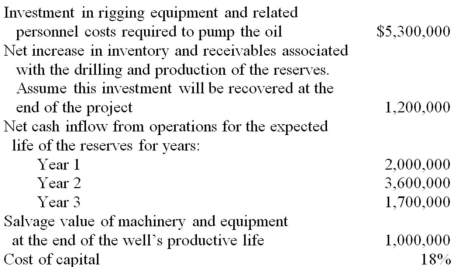(a.) Calculate the net present value of the proposed investment in the drilling and production operation. Ignore income taxes, and round answers to the nearest \$1.
(b.) What will the internal rate of return on this investment be relative to the cost of capital? Explain your answer.

64.Macy Co. is considering the investment of \$86,000 in a new machine. The machine will generate cash flow of \$18,500 per year for each year of its six-year life and will have a salvage value of \$5,000 at the end of its life. Macy Co.'s cost of capital is 10 percent.

(a.) Calculate the net present value of the proposed investment. Ignore income taxes, and round all answers to the nearest \$1.
(b.) What will the internal rate of return on this investment be relative to the cost of capital? Explain your answer.

65.The following data have been collected by capital budgeting analysts at Erica, Inc. concerning a new product line currently under consideration by management: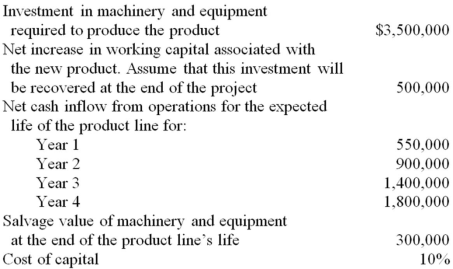(a.) Calculate the net present value of the proposed investment in the new product line. Ignore income taxes, and round all answers to the nearest \$1.
(b.) What will the internal rate of return on this investment be relative to the cost of capital? Explain your answer.

66.Delta, Inc. is considering the investment of \$75,000 in a new machine. The machine will generate cash flow of \$16,800 per year for each year of its seven-year life and will have a salvage value of \$12,000 at the end of its life. Delta Inc.'s cost of capital is 14% percent.

(a.) Calculate the net present value of the proposed investment. Ignore income taxes, and round all answers to the nearest \$1.
(b.) What will the internal rate of return on this investment be relative to the cost of capital? Explain your answer.

67.Lynn Co. is considering the investment of \$90,000 in a new machine. The machine will generate cash flow of \$10,000 per year for each year of its 15 year life and will have a salvage value of \$5,000 at the end of its life. Lynn Co.'s cost of capital is 8%.

(a.) Calculate the net present value of the proposed investment. Ignore income taxes, and round all answers to the nearest \$1.
(b.) Calculate the present value ratio of the investment.
(c.) What will the internal rate of return on this investment be relative to the cost of capital? Explain your answer.
(d.) Calculate the payback period of the investment.

68.The following data have been collected by capital budgeting analysts at Halda, Inc. concerning an investment in an expansion of the company's product line. Analysts estimate that an investment of \$210,000 will be required to initiate the project at the beginning of 2013. Estimated cash returns from the new product line are summarized in the following table; assume that the returns will be received in lump sum at the end of each year.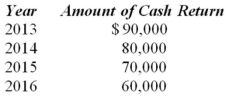The new product line will also require an investment in working capital of \$30,000; this investment will become available for other purposes at the end of the project. Salvage value of machinery and equipment at the end of the product line's life is expected to be \$20,000. The cost of capital used in Hilda, Inc.'s capital budgeting analysis is 10%.

(a.) Calculate the net present value of the proposed investment. Ignore income taxes, and round all answers to the nearest \$1.
(b.) Calculate the present value ratio of the investment.
(c.) What will the internal rate of return on this investment be relative to the cost of capital? Explain your answer.
(d.) Calculate the payback period of the investment.

69.Digital Devices, Inc. has received a special order to manufacture 10,000 CD ROM drives for an Italian computer manufacturer. Digital determines that the order will not affect its current domestic sales of CD ROM drives and because of the special nature of the order no sales commission would be paid. However, to process the order for export, an additional handling cost of \$10 per unit is estimated. The order indicates that the price of the drives cannot exceed \$200.

The company has the capacity to produce 100,000 units annually but is currently operating at 75% of available capacity. Unit selling price and costs, based on estimated actual capacity being utilized, are as follows: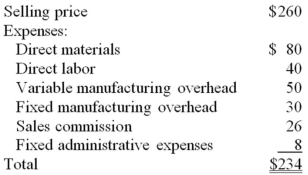(a.) Prepare a relevant cost analysis showing the effect on profit if the company accepts the special order.
(b.) How would your analysis change if Digital Devices, Inc., was producing and selling 100,000 units annually?

70.SOFT Micro Co., sells part #1973 for \$240 per unit and the standard cost of producing each unit of part #1973 is determined as follows: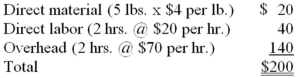SOFT received a special order for 1,000 units of part #1973. The only additional cost to SOFT is a special packaging requirement that would cost \$10 per unit.

(a.) If SOFT were currently able to sell all of its production of part #1973, what would be the minimum sales price that SOFT should consider for this special order?
(b.) Assume that SOFT has enough idle capacity to produce 1,000 units of part #1973 and that overhead is 20% variable. If SOFT wants to increase its operating profit by \$110,000, what price per unit would SOFT charge for the special order?

16-1

## Solution 5 (1 Ratings )

Solved
Accounting 1 Month Ago 536 Views